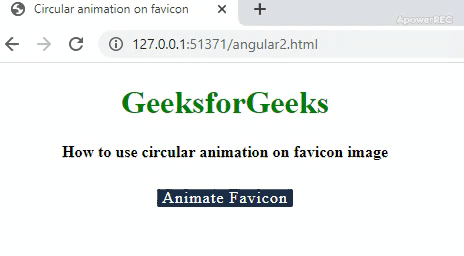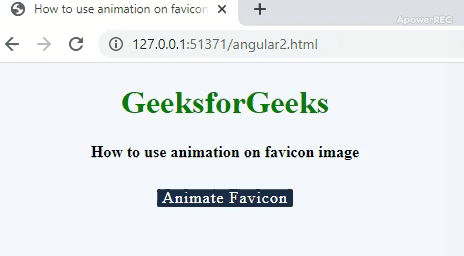# How to use animation on favicon image ?

• Last Updated : 31 Jan, 2020

A favicon is a special icon that appears at the top left corner near the web address bar. The file type can be of any jpg, png, gif or icon(.ico) image. The default favicon name is favicon.ico. The favicons are also commonly known as a shortcut icon, bookmark icon or website icon. They provide convenience to users for locating your web page. The favicons are included for a branded professional look with a specific logo that maintains a uniformity through all the pages of the website. The icons help the website in online branding. An animated favicon is created from an animated image. It is set through link tag. It is basically a set of images running one after the other within a specific time frame.

Syntax:

• To add gif as favicon:
`<link rel="icon" href="geeksforgeeks.org/favicon.gif" type="image/gif" />`
• To add normal image as favicon:
```<link rel="icon" href="favicon.ico" type="image/x-icon"/>

Note: Animated images of type GIF will work in Firefox browser. The .ico is the standard format which works well on all browsers but many websites prefer images of type PNG.

Approach: In the following example codes, the approach taken is display of progressing animation with the help of HTML canvas, javascript and mathematical geometry. An Animate Favicon button is added in the HTML page, which helps in starting the animation effect on click event. Once the drawing is done in the canvas, it is converted to a PNG image before being assigned as a favicon.

• Example 1: The following example code will show the implementation of circular animation on favicon image.

 ```<``html` `lang``=``"en"``>`` ` `<``head``>`` ` `    ``<``meta` `charset``=``"UTF-8"``>``    ``<``title``>Circular animation on favicon``    ``<``link` `rel``=``"shortcut icon"` `href``=``"gfgFavicon.png"` `width``=``32px``>``    ``<``script` `language``=``"javascript"``>``        ``onload = function() {``            ``canvas = document.querySelector('#canvasId'),``                ``context = canvas.getContext('2d');``            ``if (!!context) {`` ` `                ``// The start position for drawing circle``                ``C3qpi = 1.5 * Math.PI,``                    ``tc = pct = 0,``                    ``btn = document.querySelector('#animateBtn'),``                    ``faviconLnk = document.querySelector('link[rel*="icon"]');``                ``context.lineWidth = 3;``                ``context.strokeStyle = 'green';`` ` `                ``// On page refresh, enable the button ``                ``if (btn.disabled) btn.removeAttribute('disabled');``                ``btn.addEventListener('click', function() {``                    ``tc = setInterval(drawcircularLoader, 60);``                    ``this.textContent = 'Animating';``                    ``this.style.backgroundColor = '#99999';``                    ``this.setAttribute('disabled', '');``                ``});``            ``}``        ``};`` ` `        ``function drawcircularLoader() {``            ``with(context) {``                ``clearRect(0, 0, 32, 32);``                ``beginPath();``                ``arc(8, 8, 6, C3qpi, (pct * 2 * Math.PI / 100 + C3qpi));``                ``stroke();``            ``}`` ` `            ``// Update favicon to PNG image``            ``faviconLnk.href = canvas.toDataURL('image/png');``            ``if (pct === 100) {``                ``clearInterval(tc);``                ``btn.textContent = 'Animated';``                ``btn.style.backgroundColor = 'green';``                ``return;``            ``}``            ``pct++;``        ``}``    ````    ``<``style``>``        ``body {``            ``width: 450px;``            ``height: 300px;``            ``margin: 10px;``            ``text-align: center;``        ``}``         ` `        ``h1 {``            ``color: green;``        ``}``         ` `        ``#animateBtn {``            ``background-color: #1c2e46;``            ``border-radius: 2px;``            ``border: none;``            ``color: white;``            ``font-family: inherit;``            ``font-size: inherit;``            ``padding: 0px 5px;``            ``letter-spacing: 1px;``            ``cursor: pointer;``        ``}``         ` `        ``#animateBtn:focus {``            ``outline: none;``        ``}``         ` `        ``#animateBtn[disabled] {``            ``cursor: default;``        ``}``         ` `        ``.height {``            ``height: 10px;``        ``}``    `````` ` `<``body``>``    ``<``h1``>GeeksforGeeks``    ``<``b``>How to use circular animation on favicon image``    ``<``div` `class``=``"height"``> ``    ``<``br``>`` ` `    ````    ``<``button` `id``=``"animateBtn"``>Animate Favicon ``    ``<``br``>``    ``<``br``>``    ``<``canvas` `id``=``"canvasId"` `width``=``32` `height``=``32``>```` ` ``

• Output:• Example 2: In this example, the implementation of drawing a square which transforms the favicon into a animated image.

 ```<``html` `lang``=``"en"``>`` ` `<``head``>``    ``<``meta` `charset``=``"UTF-8"``>``    ``<``link` `rel``=``"shortcut icon"` `href``=``"gfgFavicon.png"` `width``=``32px``>``    ``<``script` `language``=``"javascript"``>``        ``onload = () => {`` ` `            ``var canvas = document.getElementById("canvas");``            ``context = canvas.getContext('2d');`` ` `            ``if (!!context) {``                ``var animateBtn = document.getElementById("animateBtn");``                ``faviconVar = document.querySelector('link[rel*="icon"]');``               ` `                ``// The styles used for the square which will ``                ``// be animated in place of favicon ``                ``let linerGradient = context.createLinearGradient(0, 0, 32, 32);``                ``linerGradient.addColorStop(0, '#8be8a7');``                ``linerGradient.addColorStop(1, '#297d4c');``                ``context.strokeStyle = linerGradient;``                ``context.lineWidth = 10;``               ` `                ``// On page refresh , the button is enabled``                ``if (animateBtn.disabled) animateBtn.removeAttribute('disabled');``                ``animateBtn.addEventListener('click', function() ``                ``{``                   ` `                    ``// Variable used for drawing incrementation``                    ``n = 0,``                       ` `                        ``// Speed interval for animation ``                        ``loadingInterval = setInterval(drawSquareLoader, 80);`` ` `                    ``this.textContent = 'Animating...';``                    ``this.style.backgroundColor = '#297d4c';``                   ` `                    ``//Once the drawing is done, the button is again disabled``                    ``this.setAttribute('disabled', '');``                ``});``            ``}``        ``};`` ` `        ``// Function for drawing square in canvas in a incrementing way``        ``function drawSquareLoader() {``            ``with(context) {``                    ``clearRect(0, 0, 32, 32);``                    ``beginPath();``               ` `                    ``// One fourth time taken to draw``                    ``if (n <= 25) {`` ` `                        ``moveTo(0, 0);``                        ``lineTo((32 / 25) * n, 0);``                    ``}``               ` `                    ``// Between second quarter of drawing``                    ``else if (n > 25 && n <= 50) {`` ` `                        ``moveTo(0, 0);``                        ``lineTo(32, 0);``                        ``moveTo(32, 0);``                        ``lineTo(32, (32 / 25) * (n - 25));``                    ``}``               ` `                    ``// Between third quarter of drawing``                    ``else if (n > 50 && n <= 75) {`` ` `                        ``moveTo(0, 0);``                        ``lineTo(32, 0);``                        ``moveTo(32, 0);``                        ``lineTo(32, 32);``                        ``moveTo(32, 32);``                        ``lineTo(-((32 / 25) * (n - 75)), 32);``                    ``}``               ` `                    ``// Between  last quarter``                    ``else if (n > 75 && n <= 100) {`` ` `                        ``moveTo(0, 0);``                        ``lineTo(32, 0);``                        ``moveTo(32, 0);``                        ``lineTo(32, 32);``                        ``moveTo(32, 32);``                        ``lineTo(0, 32);``                        ``moveTo(0, 32);``                        ``lineTo(0, -((32 / 25) * (n - 100)));``                    ``}``               ` `                    ``//Function to draw the path``                    ``stroke();``                ``}``           ` `                ``// Assigning the drawing to favicon after ``                ``// converting into PNG image``            ``faviconVar.href = canvas.toDataURL('image/png');``           ` `            ``// After the drawing is finished ``            ``if (n === 100) {``                ``clearInterval(loadingInterval);``                ``animateBtn.textContent = 'Favicon Loaded';``                ``animateBtn.style.backgroundColor = '#bbbbbb';``                ``return;``            ``}``           ` `            ``// Increment the variable used to keep``            ``// track of drawing intervals``            ``n++;``        ``}``    ```` ` `    ``<``title``>Animation on favicon image``    ``<``style``>``        ``body {``            ``width: 450px;``            ``height: 300px;``            ``margin: 10px;``            ``text-align: center;``        ``}``        ``h1 {``            ``color: green;``        ``}``        ``html {``            ``background: #f4f6fa;``        ``}``         ` `        ``#animateBtn {``            ``background: #1c2e46;``            ``color: white;``            ``font: inherit;``            ``border: none;``            ``padding: 0px 5px;``            ``letter-spacing: 1px;``            ``cursor: pointer;``            ``border-radius: 2px;``        ``}``         ` `        ``canvas {``            ``margin: auto;``            ``display: block;``        ``}``         ` `        ``.height {``            ``height: 10px;``        ``}``    `````` ` `<``body``>``    ``<``h1``>GeeksforGeeks``    ``<``b``>How to use animation on favicon image``    ``<``div` `class``=``"height"``> ``    ``<``br``>``    ``<``button` `id``=``"animateBtn"``>Animate Favicon``    ``<``br``>``    ``<``br``>``    ``<``canvas` `id``=``"canvas"` `width``=``32` `height``=``32``>`` ` ``` ` ``

• Output:My Personal Notes arrow_drop_up